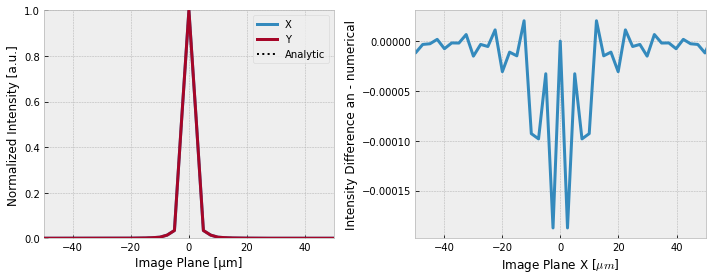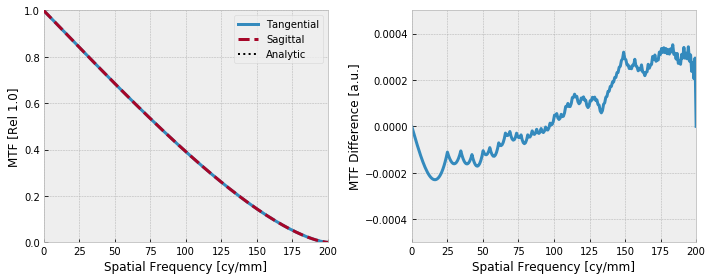Diffraction Limited PSF and MTF¶

In this example, we will show how to calculate the diffraction limited PSF and MTF for a circular aperture. We will also compare the numerically derived results from prysm with the analytical forms.

:
import numpy as np

from prysm import Pupil, PSF, MTF
from prysm.psf import airydisk
from prysm.otf import diffraction_limited_mtf

from matplotlib import pyplot as plt
%matplotlib inline
plt.style.use('bmh')
:
# system parameters
epd = 1
efl = 10
fno = efl / epd
wavelength = 0.5

# mask_target is just a performance optimization
# more samples makes for more accuracy.
# The below would get you amost the same answer:
# p = Pupil(wavelength=wavelength, dia=epd)

p = Pupil(wavelength=wavelength, dia=epd, mask_target='fcn', samples=256)
psf = PSF.from_pupil(p, efl)
u, sx = psf.slice_x

# calculate the analytical version, and the difference between the two
analytical_psf = airydisk(u, fno, wavelength)
psferr = (analytical_psf - sx)

fig, (ax1, ax2) = plt.subplots(ncols=2, figsize=(10,4))
psf.plot_slice_xy(fig=fig, ax=ax1, axlim=50)
ax1.plot(u, analytical_psf, ls=':', c='k', label='Analytic')
ax1.legend()
ax2.plot(u, psferr, lw=3)
ax2.set(xlim=(-50,50), xlabel=r'Image Plane X [$\mu m$]', ylabel='Intensity Difference an - numerical')
fig.tight_layout()One can see that the differences manifest below the fourth decimal place. It might be interesting to see the RMS error,

:
from prysm.util import rms

rms(psferr)
:
1.4915844378632847e-05

This error is over a 1D slice. If calculated over the whole image plane it would be much smaller.

Next, we consider the MTF:

:
# calculate the MTF and its error
mtf = MTF.from_psf(psf)
nu, m = mtf.tan
m_analytic = diffraction_limited_mtf(fno, wavelength, frequencies=nu)
mtferr = (m_analytic - m)

fig, (ax1, ax2) = plt.subplots(ncols=2, figsize=(10,4))
mtf.plot_tan_sag(fig=fig, ax=ax1)
ax1.plot(nu, m_analytic, ls=':', c='k', label='Analytic')
ax1.legend()
ax2.plot(nu, mtferr, lw=3)
ax2.set(xlim=(0,200), ylim=(-0.0005,0.0005), xlabel='Spatial Frequency [cy/mm]', ylabel='MTF Difference [a.u.]')
fig.tight_layout()Once again the error is at the fourth decimal place. The RMS may be interesting again,

:
rms(mtferr)
:
0.00018149825282456143